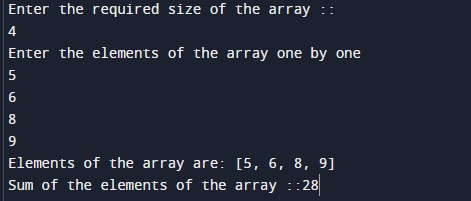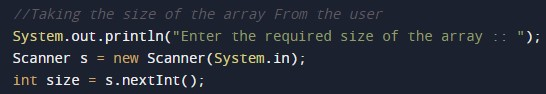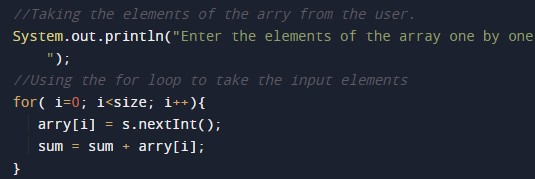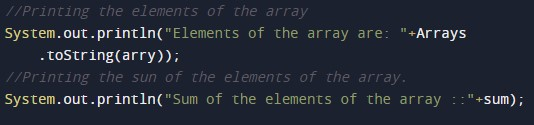# Java Program to Add the elements of an Array

In this tutorial you will learn about the Java Program to Add the elements of an Array and its application with practical example.

## Java Program to Add the elements of an Array

In this tutorial, we will learn to create a Java program that will add the elements of an Array using Java programming.

## Prerequisites

Before starting with this tutorial, we assume that you are the best aware of the following Java programming topics:

• Operators in Java Programming.
• Basic Input and Output function in Java Programming.
• Basic Java programming.
• For loop in Java programming.
• Arithmetic operations in Java Programming.

## What is An array?

The array is a collection of similar data types. An array can store multiple values with different indexes in memory by using a single variable. The array can be both single and multidimensional.

## Program description to find add the Elements of an Array.

In this program, we will first take the input array size and the elements from the user. Then we will add all the elements of the array using a for-loop. At last, we will print all the elements of the array to the user.

With the help of this program, we can add the Elements of an Array.

## Output:-In the above program, we have first initialized the required variable.• arry[] = it will hold the integer value of the input elements.
• i = it will hold the integer value.
• Size = it will hold the integer value of the input.

Input size of the array from the user.Taking the elements of the array from the user.Program Logic Code for the adding of the elements of the array.Printing output array after adding the element to the array.In this tutorial we have learn about the Java Program to Add the elements of an Array and its application with practical example. I hope you will like this tutorial.